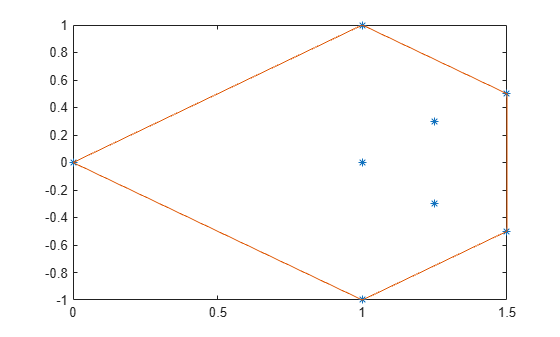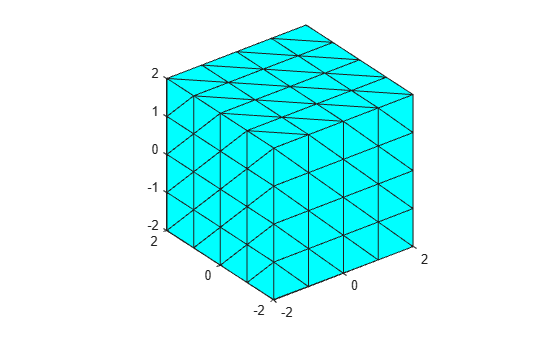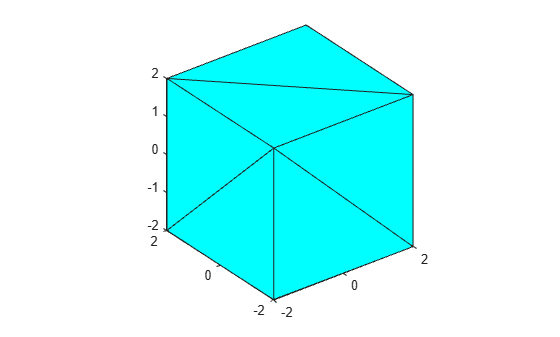Documentation

# convhull

## Syntax

``k = convhull(P)``
``k = convhull(x,y)``
``k = convhull(x,y,z)``
``k = convhull(___,'Simplify',tf)``
``[k,av] = convhull(___)``

## Description

example

````k = convhull(P)` computes the 2-D or 3-D convex hull of the points in matrix `P`.```
````k = convhull(x,y)` computes the 2-D convex hull of the points in column vectors `x` and `y`.```

example

````k = convhull(x,y,z)` computes the 3-D convex hull of the points in column vectors `x`, `y`, and `z`.```

example

````k = convhull(___,'Simplify',tf)` specifies whether to remove vertices that do not contribute to the area or volume of the convex hull. `tf` is `false` by default.```

example

````[k,av] = convhull(___)` also computes the area or volume of the convex hull.```

## Examples

collapse all

Create a matrix of 2-D points. Compute the convex hull and its area.

```P = [0 0; 1 1; 1.5 0.5; 1.5 -0.5; 1.25 0.3; 1 0; 1.25 -0.3; 1 -1]; [k,av] = convhull(P);```

Plot the original points and the convex hull.

```plot(P(:,1),P(:,2),'*') hold on plot(P(k,1),P(k,2))```Display the area.

`av`
```av = 1.7500 ```

Simplify a 3-D convex hull by removing points that do not affect its volume.

Create a set of 3-D points. Compute the convex hull and its volume. Plot the convex hull.

```[x,y,z] = meshgrid(-2:1:2,-2:1:2,-2:1:2); x = x(:); y = y(:); z = z(:); [k1,av1] = convhull(x,y,z); trisurf(k1,x,y,z,'FaceColor','cyan') axis equal```Compute and plot a simplified version of the convex hull that contains fewer points, but preserves the volume.

```[k2,av2] = convhull(x,y,z,'Simplify',true); trisurf(k2,x,y,z,'FaceColor','cyan') axis equal```Display the volumes of both convex hulls. The volumes are the same, but the simplied convex hull uses fewer points.

`av1`
```av1 = 64.0000 ```
`av2`
```av2 = 64 ```

## Input Arguments

collapse all

Points, specified as a matrix whose columns are the x-coordinates, y-coordinates, and (in three dimensions) z-coordinates.

x-coordinates, specified as a column vector.

y-coordinates, specified as a column vector.

z-coordinates, specified as a column vector.

Simplify indicator, specified as a numeric or logical `0` (`false`) or `1` (`true`).

## Output Arguments

collapse all

Indices, returned as a vector or matrix.

• For 2-D points, `k` is a column vector containing the row indices of the input points that make up the convex hull, arranged counterclockwise.

• For 3-D points, `k` is a 3-column matrix representing a triangulation that makes up the convex hull. Each row represents a facet of the triangulation. The values represent the row indices of the input points.

Area or volume of the convex hull, returned as a scalar.